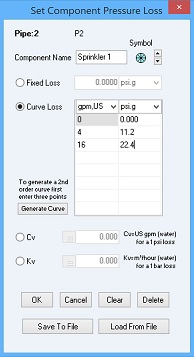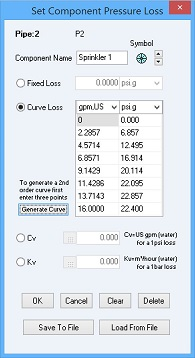## How do I model a sprinkler system with Pipe Flow Expert?

METHOD ONE – QUICK CHECK

This approach is a "quick check" approach, which uses some iteration. It is possible to define the nodes within the system, where the sprinklers are located, as "join points" and then set a demand flow at those nodes to be the flow rate that is needed through the sprinkler. Then, by using a pump set to "Fixed Head" mode, the pump head can be adjusted as required, in order to obtain the required pressure at each of the nodes in the system where the sprinklers are located. If the pressure that is needed by the sprinkler is not being met at each of these nodes, an increase in the pump head can be applied, and the system re-solved to see how much the increase in pump head has increased the pressure at the nodes representing the sprinklers. (N.B. it is not possible to run the pump in "Fixed Flow Rate" mode using this approach, as there would then be no pressure reference for the system).

Once the required pressures have been achieved at the sprinklers, then a suitable pump can be selected that can deliver the required pump head for the defined flow rate, and the "Fixed Head" pump can be removed. The system can then be solved using the selected pump, and the pump's operating point will be calculated such that it meets the defined flow rate requirement.

Pipe Flow Expert example systems "Isometric_05_Water_Distribution_Network" and "Isometric_06_Water_Circulation_System" provide illustrations of specifying a demand flow.

METHOD TWO – ACCURATE SOLUTION

This second, accurate, method models the performance of the sprinkler and will calculate the exact flow rate out of each sprinkler. In a real-world system, the flow out of each sprinkler will be slightly different depending on its position and pressure loss through the network of pipes.The approach is to use a Component and an end-pressure node to model the sprinkler. In this approach, a flow versus pressure-loss curve for the component is specified that represents the performance characteristic of the sprinkler (this can be a set of flow versus pressure points or this can be specified via Sprinkler K value). A pump is then defined, which can be set to run in “Fixed Flow Rate” mode, or alternatively a pump with an appropriate performance curve can be selected that will run in "Set Speed" mode. If using a "Fixed Flow Rate" pump, then Pipe Flow Expert will calculate the pump head required to achieve the specified flow rate. If using a pump running in "Set Speed" mode, then the operating point for the pump that defines the pump head added and the associated flow rate, will be calculated. For either pump modelling approach, the system will be balanced using the appropriate flow/pressure loss point on the Component pressure-loss curve that corresponds to the flow rate through the system.

The following explains how to model the sprinklers using Pipe Flow Expert Components.

USING A COMPONENT

To model the flow versus head loss through a sprinkler in a Pipe Flow Expert model you can use a component object, specifying the imperial Sprinkler K value or metric Sprinkler K value (where this represents the flow versus pressure loss performance characteristic of the sprinkler - the sprinkler manufacturer will often provide this value).Alternately, to model the flow versus head loss through a sprinkler in a Pipe Flow Expert model you can use a component object with a data table of flow rates versus pressure losses that define the performance characteristic of the sprinkler. You only need to enter three points into the component data table & then click the “Generate Curve Loss” button to fill the data table with a set of points which lie on the flow versus pressure loss curve.

As an example, we could enter the following three US gpm and psi data points into the table on the "Set Component Pressure Loss" screen:then click "Generate Curve". The generated curve would then contain a list of equally spaced flow-rate data points between 0 and 16 US gpm with the corresponding pressure loss for each flow rate point:You can use a Component on a pipe in Pipe Flow Expert to model the Sprinkler performance information, and the software will balance and solve the system, finding the operating flow rate and pressure loss through the component when taking account of all the other flow and pressures in the system.

Secure Online PaymentsPipe Flow Software: Piping design, Pressure drop calculator, Flow rate calculator, Pump head calculations, Pump selection software.  Copyright © Pipe Flow Software 1997-2020